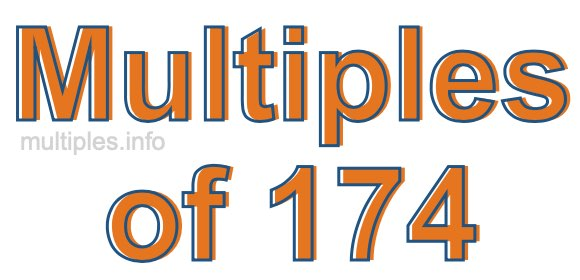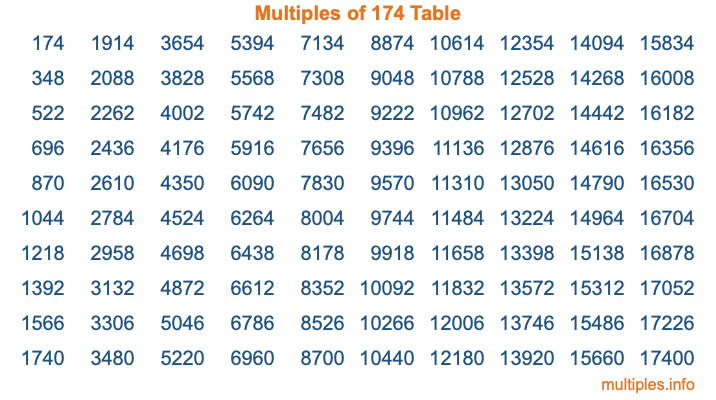Multiples of 174Welcome to the Multiples of 174 page. Here we will first teach you everything you will ever need to know about the multiples of 174, and then give you a study guide summary of everything we taught you to make sure you remember it all. Use this page to look up facts and learn information about the multiples of 174. This page will make you a multiples of one hundred seventy-four expert!

Definition of Multiples of 174
Multiples of 174 are all the numbers that when divided by 174 equal an integer. Each of the multiples of 174 are called a multiple. A multiple of 174 is created by multiplying 174 by an integer.

Therefore, to create a list of multiples of 174, you start with 1 multiplied by 174, then 2 multiplied by 174, then 3 multiplied by 174, and so on for as long as you want. Thus, the list of the first five multiples of 174 is 174, 348, 522, 696, and 870. To see a larger list of multiples of 174, see the printable image of Multiples of 174 further down on this page. We also have a category where you can choose any nth multiple of 174.

Multiples of 174 Checker
The Multiples of 174 Checker below checks to see if any number of your choice is a multiple of 174. In other words, it checks to see if there is any number (integer) that when multiplied by 174 will equal your number. To do that, we divide your number by 174. If the the quotient is an integer, then your number is a multiple of 174.

Is  a multiple of 174?

Least Common Multiple of 174 and ...
A Least Common Multiple (LCM) is the lowest multiple that two or more numbers have in common. This is also called the smallest common multiple or lowest common multiple and is useful to know when you are adding our subtracting fractions. Enter one or more numbers below (174 is already entered) to find the LCM.

Check out our LCM Calculator if you need more details about the Least Common Multiple or if you need the LCM for different numbers for adding and subtraction fractions.

nth Multiple of 174
As we stated above, 174 is the first multiple of 174, 348 is the second multiple of 174, 522 is the third multiple of 174, and so on. Enter a number below to find the nth multiple of 174.

th multiple of 174

Multiples of 174 vs Factors of 174
174 is a multiple of 174 and a factor of 174, but that is where the similarities end. All postive multiples of 174 are 174 or greater than 174. All positive factors of 174 are 174 or less than 174.

Below is the beginning list of multiples of 174 and the factors of 174 so you can compare:

Multiples of 174: 174, 348, 522, 696, 870, etc.

Factors of 174: 1, 2, 3, 6, 29, 58, 87, 174

As you can see, the multiples of 174 are all the numbers that you can divide by 174 to get a whole number. The factors of 174, on the other hand, are all the whole numbers that you can multiply by another whole number to get 174.

It's also interesting to note that if a number (x) is a factor of 174, then 174 will also be a multiple of that number (x).

Multiples of 174 vs Divisors of 174
The divisors of 174 are all the integers that 174 can be divided by evenly. Below is a list of the divisors of 174.

Divisors of 174: 1, 2, 3, 6, 29, 58, 87, 174

The interesting thing to note here is that if you take any multiple of 174 and divide it by a divisor of 174, you will see that the quotient is an integer.

Multiples of 174 Table
Below is an image of the first 100 multiples of 174 in a table. The table is in chronological order, column by column. The first column has the first ten multiples of 174, the second column has the next ten multiples of 174, and so on.The Multiples of 174 Table is also referred to as the 174 Times Table or Times Table of 174. You are welcome to print out our table for your studies.

Negative Multiples of 174
Although not often discussed or needed in math, it is worth mentioning that you can make a list of negative multiples of 174 by multiplying 174 by -1, then by -2, then by -3, and so on, to get the following list of negative multiples of 174:

-174, -348, -522, -696, -870, etc.

Multiples of 174 Summary
Below is a summary of important Multiples of 174 facts that we have discussed on this page. To retain the knowledge on this page, we recommend that you read through the summary and explain to yourself or a study partner why they hold true.

There are an infinite number of multiples of 174.

A multiple of 174 divided by 174 will equal a whole number.

174 divided by a factor of 174 equals a divisor of 174.

The nth multiple of 174 is n times 174.

The largest factor of 174 is equal to the first positive multiple of 174.

174 is a multiple of every factor of 174.

174 is a multiple of 174.

A multiple of 174 divided by a divisor of 174 equals an integer.

174 divided by a divisor of 174 equals a factor of 174.

Any integer times 174 will equal a multiple of 174.

Multiples of a Number
Here you can get the multiples of another number, all with the same attention to detail as we did for multiples of 174 on this page.

Multiples of
Multiples of 175
Did you find our page about multiples of one hundred seventy-four educational? Do you want more knowledge? Check out the multiples of the next number on our list!